You are here: Start » AVL.NET » Geometry 3D Features

# Geometry 3D Features

Icon Name Description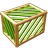Box3DCenter Returns the center of the input box in 3D.Box3DCharacteristicPoint Returns a characteristic point of a box in 3D.Box3DSurfaceArea Returns the surface area of the input box in 3D.Box3DVolume Returns the volume of the input box in 3D.Boxes3DBoundingBox3D_OrNil Computes the bounding box 3D of given boxes in 3D; returns NIL if the array is empty.BoxesBoundingBox3D Computes the bounding box 3D of given boxes in 3D.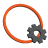Circle3DArea Computes the area of a circle in 3D.Circle3DBoundingBox Computes the smallest box in 3D containing a circle in 3D.Circle3DPerimeterLength Computes the length of a circle in 3D perimeter.Plane3DNormalVector Computes the normal vector of a line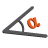Plane3DOrientation Computes the orientation of a plane as angles in the range from 0 to 180 between the plane and coordinate planes.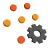PointsBoundingBox3D Computes the smallest box in 3D containing an array of points in 3D.PointsBoundingBox3D_OrNil Computes the smallest box in 3D containing an array of points in 3D; returns NIL if the array is empty.PointsMassCenter3D Computes the central point of the input points.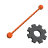Segment3DBisector Computes a plane passing through the center of a segment in 3D at a right angle.Segment3DBoundingBox Computes the smallest box in 3D containing a segment in 3D.Segment3DCenter Computes the center point of a segment in 3D.Segment3DLength Computes the length of a segment in 3D.Segment3DLine Computes the line in 3D passing through a segment.Segment3DVector Returns the vector [x2 - x1, y2 - y1, z2 - z1]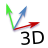Vector3DAzimuth Computes the angle between a 3D vector's projection to the XY plane and the X axis measured toward Y axis, as an angle in the range from 0 to 360.Vector3DElevation Computes the angle between a 3D vector's projection to the XY plane and itself measured toward Z axis, as an angle in the range from -90 to 90.Vector3DLength Computes the length of a 3D vector.